# The Lemniscates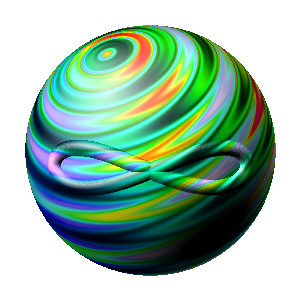The denomination "lemniscate" derivates out of Latin and means: chain link. A smith, who forges a chain, whacks the round chain links into an oval and twist them a bit, for the sake, that the chain gets longer and behaves more like a rope.
As lying eight the lemniscate has also the meaning of infinity, because it has no start and no end, but in the middle something happens.

all nine!with the same pole angle

For my windmills I searched a way, how I could transform a circular movement into a (spheric) lemniscate. The plane three known lemniscates

• the lemniskate of Bernoulli
• the lemniskate of Booth
• the lemniskate of Gerono

are not good for my purpose. I first found the Cardan-Lemniskate. Virtually this one would have been sufficient, but the mathematics behind let me not go. And so I found next the Basislemniscate. After that I found also the Double-Lemniscate. And after that I gave them numbers. Now there are countable nine.

Now I found a way how I could vary the opening angle of the lemniscate fluent from 0° to θ.

A possible mathematical definition of a lemniscate sounds like that:
A lemniscate is the closed curve of a point in a 2-dimensional Riemann manifold, whose continuously diversifying intrinsic curvature runs through 4 symmetry-phases. At the end of the first quarter phase the curvature gets zero, in the second quarter it is point-symmetric towards the place, where it was zero. The last two quarters are axle-symmetric mirrored towards the both first quarters (just like a cosine curve).

In terms of mathematics:
Be f: [0, T] → ℝ³ a curve upon the plane g: (u, v) → ℝ³ and K(t) ≥ 0, for t ∈ [0, T/4[ and K(T/4) = 0, than is valid for the intrinsic curvature k i of the curve:

k i(t) = {K(t),            for t ∈ [0, T/4[,
-K(T/2-t),   for t ∈ [T/4, T/2[,
-K(t-T/2),   for t ∈ [T/2, 3*T/4[,
K(T-t),        for t ∈ [3*T/4, T]}

and f(0) = f(T)

This definition has the advantage, that you can use it also on curved surfaces like tori, paraboloids and so on. Which properties an area must have, that you are after the 4 phases at the starting place again? Well, this is another question...

(The intrinsic curvature k i is the curvature of a curve of the area. For a bicyclist the curvature is the posture of the handlebars. If he drives upon the equator-circle of a ball, the handlebars are straight line, therefore the intrinsic curvature is k i = 0. Does he drive the same circle upon a plane, the intrinsic curvature is the curvature of the circle in the plane k i = 1 / r. If you want to calculate the intrinsic curvature of the curve upon an area, you must lay the sliding-plane at the points of the curve along the plane. The sliding-plane is spanned by the tangent-vector and the main-perpendicular-vector of the curve. In this sliding-plane you get the searched curvature by using the theorem of Meusnier.)

In distinction towards the lemniscates of Bernoulli, Booth and Gerono of the plane, I need lemniscates on the curvatured surface of a ball for my windmills and for that sake the definition of a lemniscate have to be more common but have to contain the three named lemniscates as a special case.

Analogue to this I can deliver three different definitions of a circle:
A circle is

• the set of all points, that have towards a point of the area the same distance   (point-definition)
• the curve of a point, that have everywhere the same intrinsic curvature   (line-definition)
• the curve of a point with given length, that divides the area in two part-areas, whereas the quotient of lower to greater area is maximal   (area-definition)

In the case of a plane all three definitions are equivalent.

On a paraboloid for example there are three different types of circles with Euclidean distance- and area-norm. (Euclidean distance-norm: dr² = dx² + dy², Euclidean area-norm: dA = dx * dy; with dx ⊥ dy.) On a curved surface beside the ball, the circle is dependent of the place and the definition. The point-definition sounds most logic, because everybody associates a radius with a circle. The radius follows a geodesian-line, which comes from the middle-point. Definition 1 and 2 are mathematical not equivalent, because a close spiral curve upon a torus around the middle cannot be pressed like a paraboloid.

Torus-spiral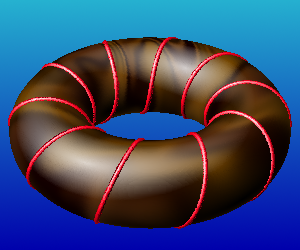the red coil is after definition 1 no, but after definition 2 a circle

Double-cone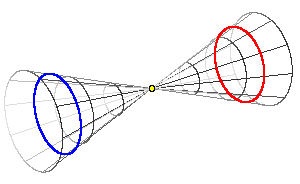The blue "circle" has the same distance towards the yellow point like the red.
Therefore it is after definition 1 only a demi-circle.

## The particular lemniscates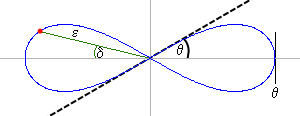The cardan-lemniscate with the relevant angles

Cardan-lemniscate
In polar coordinates you can write the cardan-lemniscate most easily. Then it is:

cos(θ) = cos(ε) ∙ cos(δ)

(You get the same formula for the perpendicular spheric triangle with θ as hypotenuse.)

The angle θ is the angle under which the lemniscate cuts the abscissa. The angle ε is the pol-angle and the angle δ is the meridian.

The area of the cardan-lemniscate upon the surface of the unit ball is:

F = 4∙(θ - cos(θ)∙ln(1/cos(θ) + tan(θ)))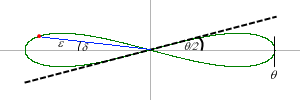The basis-lemniscate with the relevant angles

Basis-lemniscate
The basis-lemniscate got its name because it is invariant towards deferrals of angles. It cuts the abscissa with the angle θ/2 and has got this polar coordinates:

cos(θ/2) = cos(ε/2) ∙ cos(δ)

The area of the basis-lemniscate upon the unit ball is:

F = 4∙(θ - sin(θ))

(You get the same formula for the x-coordinate of the cycloide in parametric representation.)The double-lemniscate with the relevant angles

Double-lemniscate
This one is a bit more complicated. In polar coordinates:

cos(θ)/sqrt(2) = cos(arccot(cos(ε))) ∙ cos(δ)

Fortunately nobody needs its area.

No. 4 to No. 9
The polar coordinates of the other lemniscates? Oh, yes, oh... I mean that I already have understood this question...

## The Analemma of Vitruv

I could show, that the Analemma of Vitruv is a displaced basis-lemniscate. You get the analemma, if you look for one year exactly each day 24.00 hours later at the same place to the sky and you mark the position of the sun on a screen.

The analemma of the earth at the morning sky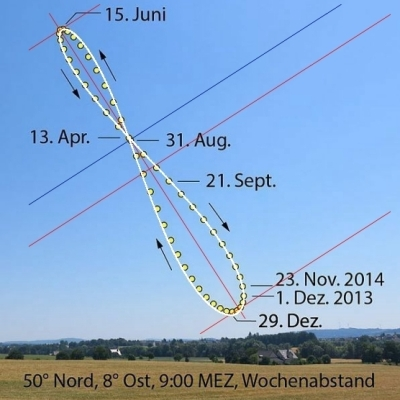the picture is a processing from wikipedia

It was a tremendous performance to find out the difference in the lengths of the days with the resources of the ancient time.

The calculation of Vitruv is unusable indeed.

## Applications of the lemniscates

• Mainly for the Zédolille Windmill of course. By moving oblique behind, the plane moves perpendicular to the virtual wind and the virtual wind is decisively.

• The inversion gives a new propulsion for ships with less cavitation and less loss of energy due to the swirl of the water behind a propeller.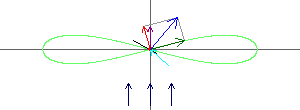the lemniscate as a windmill with the vectors